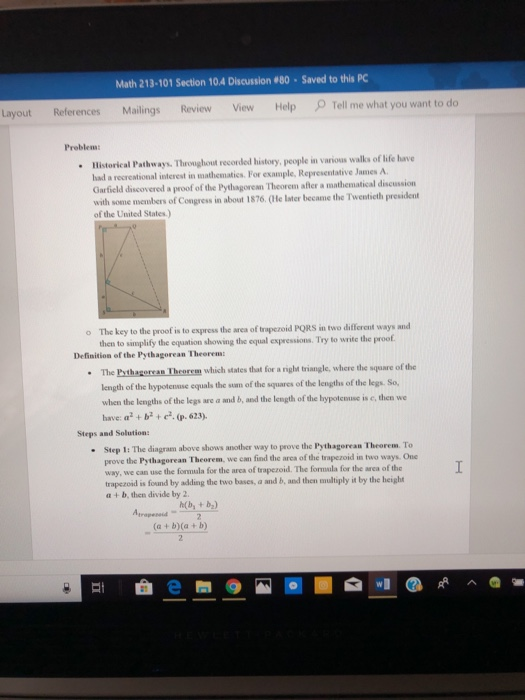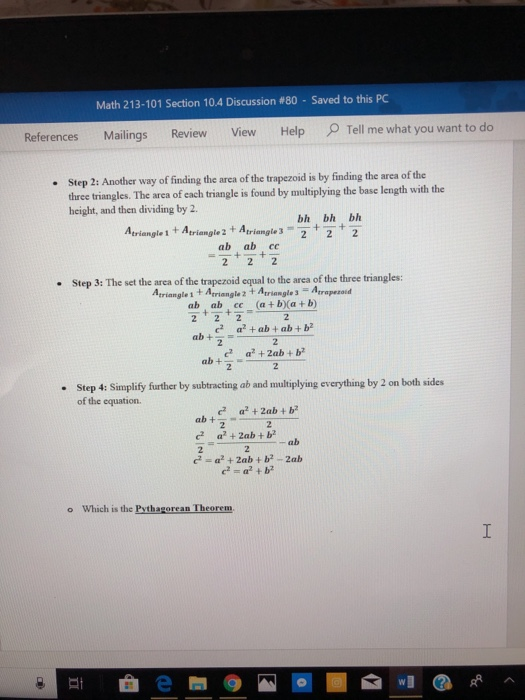Question

# For this i presented problem number 80 from section 10.4. i need to answer the following question...

for this i presented problem number 80 from section 10.4. i need to answer the following questions in the third picture. just those questions. no cursive please its hard to read.Math 213-101 Section 10.4 Discussion #80-Saved to this PC Layout References Mailings Review View Help Tell me what you want to do Problem: Historical Pathways. Throughout recorded history, people in various walls of life have had a recrentional interest in nathematics. For example, Represenlative James A Garfield discovered a proof of the Pythagorean Theorem after a mathematical discussion with some members of Congress in about 1876. (le lster became the Twenticth president of the United States.) The key to the proof is to express the arca of trapezoid PORS in two different ways then to simplify the equation showing the equal expressions. Try to write the proof o Definition of the Pythagorean Theoren The Psthagorcan Theoren which states that for a right triangle, where the square of the length of the hypotenuse equals the suam of the squares of the lengths of the legs. So, when the lengths of the legs are a and b, and the length of the bypotcnuse is c, then we have: a +b + c. (p. 623) Steps and Solution: Step 1: The diagram above shows another way to prove the Pythagorean Theorem. To prove the Pythagorean Theorem, we can find the area of the trapezoid in two ways. One way, we can use the trapezoid is found by adding the two bases, a and b, and then naltiply it by the height a + b, then divide by 2 - formala for the arca of trapezoid. The formula for the area of tho Atrapereid (a+ b(a+b
. Math 213-101 Section 104 Discussion #80-Saved to this PC References Mailings Review View Help Tell me what you want to do Step 2: Another way of finding the area of the trapezoid is by finding the area of the Atriangle 1 +Ariangle 2 + Atri222 Step 3: The set the area of the trapezoid equal to the area of the three triangles: three triangles. The arca of each triangle is found by multiplying the base length with the height, and then dividing by 2. bh bh bh ab ab ce Atriangle 1 + Atriangle 2+ Ariangle3Atrapezod ab ab ce at ba+ b a +Zab+b Step 4: Simplify further by subtracting ab and multiplying everything by 2 on both sides of the equation. a2 +2ab + b2 c2 ab+ 22 c2 a2 +2ab + b2 2ab o Which is the Pythagorean Theorem
I already did the problem, need to do a reflection on my work that I attached below.I need to answer this: Do you think you could have solved the problem differently? s there any other problem-solution strategy that might have worked? What did you learn from presenting the problem? What was the point of the problem itself? Which problem in the textbook does it relate too?

1.) This is the best approach to solve this problem or i would rather say the traditional approach. I would have solved in the same manner.

2.) No.

3.) By presenting this problem we get to learn about the ways to find area of the trapezoids and the triangle and verify it using the Pythagoras theorem.

4.) The point was to verify the Pythagoras theorem.

#### Earn Coins

Coins can be redeemed for fabulous gifts.